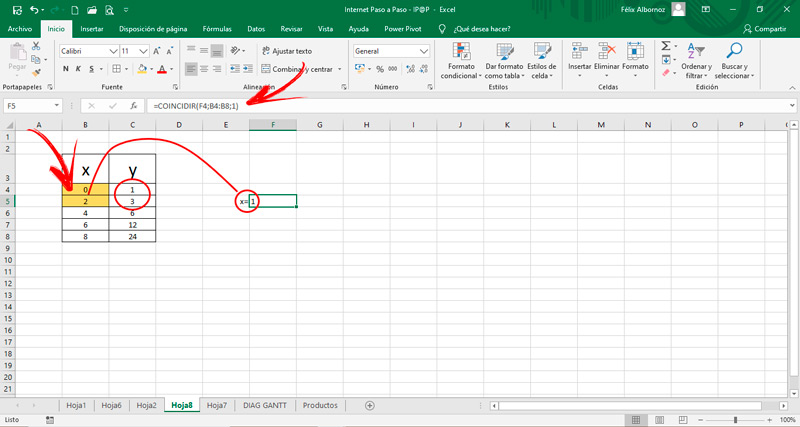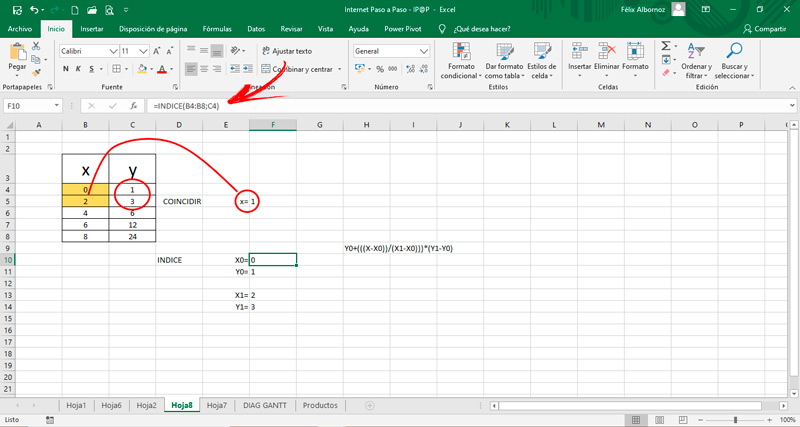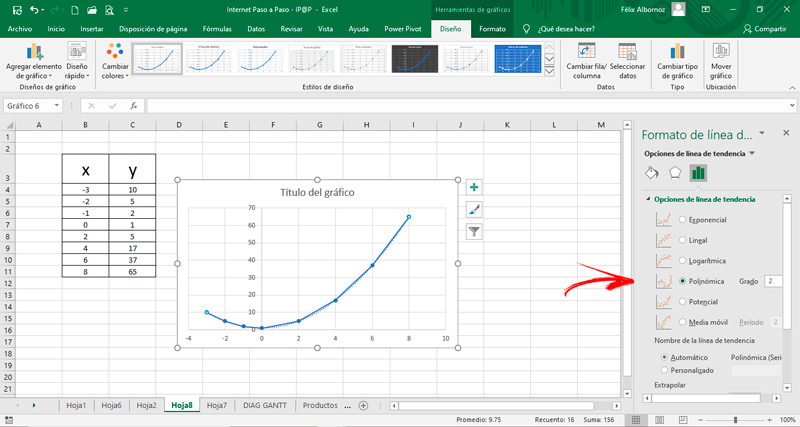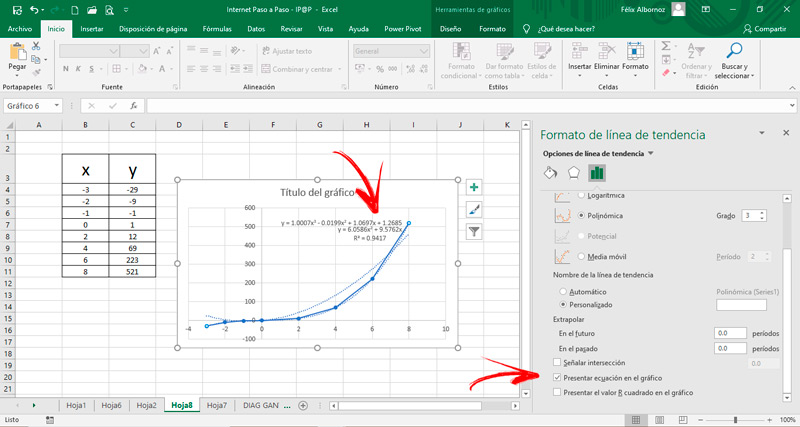Interpolate in Microsoft Excel  Step by Step Guide ▷ 2020

The degree of scope that Excel has, to study known relationships and work with functions, is very wide. Therefore, many people do not know all the power that this calculation tool.

A somewhat complicated task is to estimate values ​​based on known intervals. That is, establish as precisely as possible, what is the number taking as reference certain values.

Because of this, there is the data interpolation technique, which we will talk about in the following paragraphs. We will teach you the steps you will take to estimate values ​​quickly and safely. Don’t miss out on anything.

What is a data interpolation and what is it for in my spreadsheet?

Data interpolation is a mathematical tool that It is used to estimate data that are within a known range. By knowing the extreme values, you can interpret those that exist within that set. The opposite of interpolation is known as extrapolation and is the data found outside of rank known.

There are different methods to know the internal points. So the results will be rough estimates that will vary according to the type of method. Among the best known are linear, quadratic or parabolic interpolation and polynomials.

These methods are useful in practice for estimate values ​​from known data. For example, you can estimate the number of tourists who visited Barcelona in 2019 based on specific data obtained in 2017.

Thus, can be applied to any type of information. Either the environmental temperature in a certain area of ​​our country or Europe, the housing density, students enrolled in an educational establishment and up to amount of years of average life discriminated by gender of people.

This means that Microsoft Excel is an excellent tool to use it and obtain estimates through the data interpolation.

Types of interpolation What are all the ones that exist and can be done in Excel?

The types of interpolation that you can use in Excel to estimate values ​​within a known set are as follows:

LinearAmong the different types of interpolation that exist, the easiest method is the linear. This is because the possible solutions of the variable X it is only one. Also, joining two points in a graph, the line can now be drawn, so find the pairs X0; Y Y X1; Y1 it has fewer steps.

This does not mean that this method it is not effective nor is it much better than the others, since it is not possible to determine exactly which is better if the behavior of the curve is not taken into account.

For this function the formula is used:

• Y + {[(X-X)]/ (X1-X)]} * (Y1-Y).

You can also know the equation of the line through the following function:

• AXIS(known_y; known_x) for determine the constant a.
• PENDING(known_y; known_x) for set slope, that is the b.

Parabolic

The parabolic function or also known as quadratic or level 2 polynomial, presents a graph with a minimum or maximum and has the equation formula y = a + bx + cx2. The function is a polynomial that is used to estimate values ​​is y = a + b (xx) + c (xx) (x-x1).

To work in Excel you can replace the values ​​of the equation, or use formulas like COINCIDE and INDEX.

Polynomial Level 3

The function is used y = anxn + … + a1x + ao; with n = 3. In this way it can be determined that a is a0, a1, a2, a3 Y x is x0, x1, x2, x3.

To use Excel and establish the vector a, this formula must be created:

• = LINEST(y, x ^ (1,2,3,4))

Polynomial Level 4

Same as him level 3 polynomial you can run the “Quantity n” polynomials, so the treatment does not matter from level 3 to number n.

In this way we can conclude that the method of degree 4 has the same steps and identical characteristics to solve a value within a known interval as that of degree 3.

Steps to interpolate data or ranges in a Microsoft Excel worksheet

Then you can find the steps what should you do to interpolate data within a known range using Microsoft Excel. We will show you a guide for a linear function, a parabolic and for polynomials of level 3 and 4.

Let’s see them:

LinearTo use a linear interpolation in Excel you must divide the task into two stages.

The first thing you will have to do are these steps:

• Go to the tab “Formula” and then search the menu “Function library” the tool “Search and reference”.
• When you click on it, will open a window, you will have to choose the function “COINCIDE”. Since in this way you will find a relative position inside the matrix.
• Choose how searched value a cell in which you want to obtain the data.
• Then select matrix From the axis X.
• In match type you will have to choose number 1, since if you choose 0 it will be exact or if you choose number -1 you will have a higher value result.

The value you will get is equal to 1. This means that between the values ​​of Y correspond to the estimate you are looking for.

Now you must use INDEX formula to set the values X; Y and X1; Y1.

To do this, follow these steps:

• Use the formula INDEX (array; value of Y when it is the first term).
• This way you can get the values ​​you are looking for.
• When I have the four values you will have to replace in the formula Y + (((XX)) / (X1-X))) * (Y1-Y).
• You will get the value of Y. That means it’s the estimated value when X is between 2 values.

ParabolicWhen looking for the equation type y = a + bx + cx2 we will do it for graphic medium To find the parabolic method. You can also do it as we remove it with the MATCH function and INDEX, but we want to show you a simpler method for simple cases.

The steps to follow are those:

• Once you have entered the values from X and of Y select the matrix.
• Go to “Insert” and then click “Recommended graphics”.
• Look for the option “Dispersion (XY)” and click on it.
• Choose the graphic that suits you best.
• Right click on the line that was drawn.
• Click on the option “Add trend line …”.
• Choose the function “Polynomial” and then write “2” in the countryside “Degree:”.
• Scroll down and check the boxes “Mark intersection”, “Present equation on the graph” Y “Present the R squared value on the graph”. This will allow you to get all data What do you need to find the values ​​you want from Y.

Polynomial Level 3The steps you will have to do are the same as those shown for the parabolic function. Once you have all data loaded on a real table insert a drawing and then set the trend line.

To finish you must activate the same boxes and thus you will get the formula you need to get any value of Y.

Polynomial Level 4

The same happens with the polynomial level 2, or parabolic, and level 3 you will have to do the same steps for a level 4 function.

If you have any questions, leave them in the comments, we will answer you as soon as possible, and it will also be of great help to more members of the community. Thank you! 😉

Rate this post